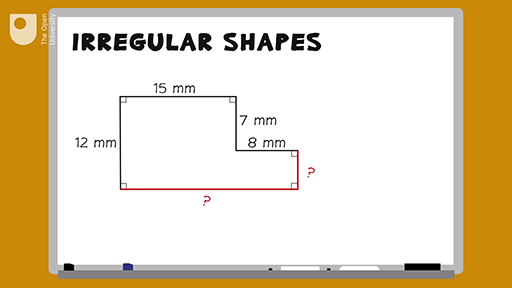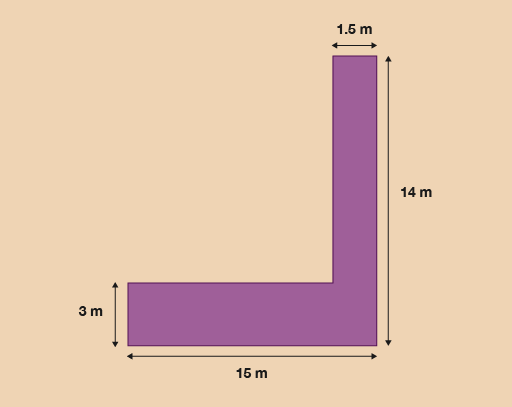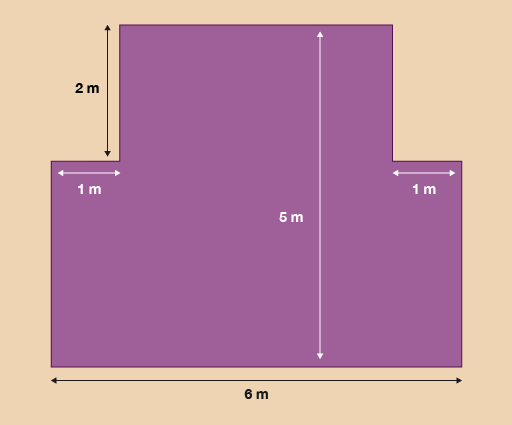Everyday maths for Health and Social Care and Education Support 1

Start this free course now. Just create an account and sign in. Enrol and complete the course for a free statement of participation or digital badge if available.

Free course

# 1.1 Measuring the perimeter of irregular shapes

## Example: How to measure the perimeter of an irregular shape

How would you measure the perimeter of an irregular shape – an L-shaped room, for instance – if you didn’t have all of the measurements that you would need? Watch the following video to find out.Skip transcript

#### Transcript

Say you needed to find the perimeter of this shape, but two of the measurements are missing. How would you find them out? You can assume that all of the corners are right angles. You need to use the measurements that you do know.

So if you want to find out the missing side on the right, you know that the left-hand side opposite to this is 12 millimetres long. And there is another measurement on the same side in the middle of the shape that is 7 millimetres long.

The missing length must then be the difference between 7 and 12 millimetres. Here’s a sum to show this: 12 minus 7 equals 5 millimetres. So the answer for the right-hand side is 5 millimetres. You can check this by adding 5 and 7, which makes 12 millimetres, the same as the left-hand side.

To find out the length at the bottom of the shape, because you know that all of the corners are right angles, you can add together the other two parallel measurements that are given: 15 plus 8 equals 23 millimetres. So the bottom of the shape is 23 millimetres.

Now you know the measurements for all of the shape’s edges, you can measure the perimeter. 12 plus 15 plus 7 plus 8 plus 5 plus 23 equals 70 millimetres. So the perimeter is 70 millimetres.

End transcript

Interactive feature not available in single page view (see it in standard view).

Now try the following activity. Remember to check your answers once you have completed the questions.

## Activity 3: Finding the perimeter

Note that you can assume that all of the corners in the images in this activity are right angles.

1. A residential care home has employed a gardener to improve the outdoor areas for the residents. The gardener has added a lily pond to the garden and decides to lay a new path next to the lily pond for residents to walk around. The drawing shows the dimensions of the path.Figure 6 A pathway

The gardener decides to paint a white line around the perimeter of the path. What is the perimeter of the path?

2. A hospital has a new extension.Figure 7 A new hospital extension

The hospital’s health and safety officer wants to attach an emergency lighting strip around the border of the floor of the building. What is the perimeter of the new extension?

1. To calculate the missing sides you should have carried out the following calculations:

• 14 – 3 = 11
• 15 – 1.5 = 13.5

Now that you have found the missing sides, you can add them all together:

• 15 + 14 + 1.5 + 3 + 13.5 + 11= 58 m
2. To calculate the missing sides you need to carry out the following calculations to calculate the perimeter:

• 5 – 2 = 3
• 6 – 2 = 4

Now that you have found the missing sides, you can add them all up together to calculate the perimeter:

• 6 + 3 + 3 + 1 + 1 + 2 + 2 + 4 = 22 m

## Summary

In this section you have learned how to work out the perimeter of both simple and irregular shapes.

FSM_SSH_1